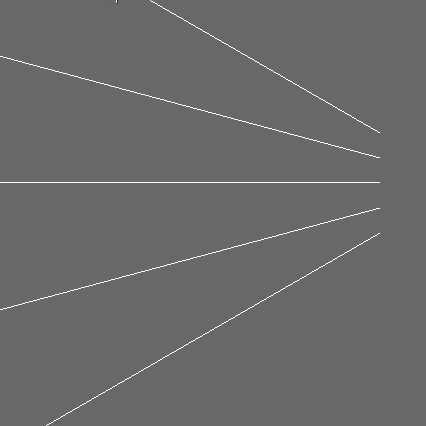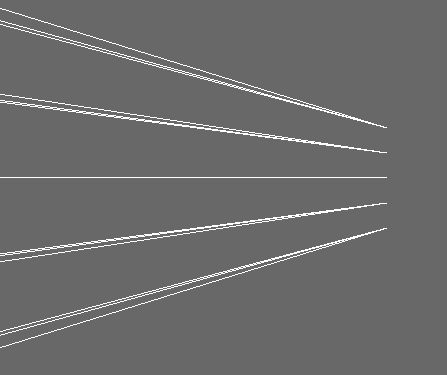# Angles to Vectors

What I’m trying to do is to project a bunch of rays from a node but I don’t want them to be parallel but to go out in a angle (like light coming from a flash-light) and I want to control both the horizontal and vertical angles (also negative angles and parallel lines at 90deg).

I tried using a LensNode, tried some trigonometry but I had little success, something was always wrong. My last attempt was to make a quaternion, set its heading and pitch then get its forward vector, it would be quite elegant and simple… if it worked. I’m not sure if I’m doing something wrong, or if it’s some inherent property of quaternions but the vector I get is just slightly wrong (at least in one plane):
This is how I expect it to look:(this is from a orographic camera, there are 5 rows and 5 columns of rays, but the rays overlap all nice)
…and this is what I get:Here is my code:

``````from panda3d.core import *
from direct.showbase import ShowBase
from direct.showbase.DirectObject import DirectObject
from direct.gui.OnscreenText import OnscreenText

# Function to put instructions on the screen.
return OnscreenText(text=msg, style=1, fg=(1, 1, 1, 1), scale=.05,
pos=(0.08, -pos - 0.04), align=TextNode.ALeft)

class App(DirectObject):
def __init__(self):
self.base = ShowBase.ShowBase()
#make the camera orthographic
self.base.disableMouse()
base.camLens=OrthographicLens()
film_size=Vec2(base.get_size())
film_size/=film_size/16 #zoom out
base.camLens.set_film_size(film_size)
base.cam.node().set_lens(base.camLens)
#instructions
self.inst1 = addInstructions(0.06, "UP ARROW: View from top")
self.inst2 = addInstructions(0.12, "RIGHT ARROW: View from side")
#view from the side
self.set_view((-100, 0, 0), (-90, 0, 0), 'side')

node=render.attach_new_node('node')
rows=5
columns=5
row_offset=0.5
column_offset=0.5
row_angle=30.0
column_angle=15.0
lines=[]
q=Quat()
for row in range(rows):
for column in range(columns):
local_origin=Point3(row*row_offset, 0, column*column_offset)
origin=render.get_relative_point(node, local_origin)
#scale the angles
hpr=(row_angle-row_angle*row/(rows-1)*2.0,
-1.0*(column_angle-column_angle*column/(columns-1)*2.0),
0.0)
q.set_hpr(hpr)
v=q.get_forward()*100.0 #target a point 100.0 units away
target=render.get_relative_vector(node, v)+origin
lines.append((origin, target))
#draw lines
l=LineSegs()
for origin, target in lines:
l.move_to(origin)
l.draw_to(target)
line=render.attach_new_node(l.create())
#key binds
self.accept('arrow_right', self.set_view, [(-100, 0, 0), (-90, 0, 0), 'side'])
self.accept('arrow_up', self.set_view, [(0, 0, 100), (-90, -90, 0), 'top'])

def set_view(self, pos, hpr, name):
'''Sets the camera hpr and pos andl also display a name'''
base.camera.set_pos(pos)
base.camera.set_hpr(hpr)
self.inst3.destroy()
self.inst3 = addInstructions(0.18, "Current view: "+name)
#run it
app=App()
app.base.run()
``````

If someone has any insights how to fix this, or some other idea how to get the same result please do help.

The reason it looks correct from the top, but wrong from the side, is because of the order in which component angles are applied when calculating an orientation: first the roll is applied, followed by the pitch and finally the heading. Since the heading (the component angle in the horizontal plane) is applied last, your solution looks correct when looking at the rays from above (top view).
However, you use the same hpr for the vertical angles as well, but you would need an angle where the pitch is applied last, so it doesn’t work. The solution is to replace your quaternion with two different quaternions, one describing only the heading and a second quaternion describing only the pitch. Then you can multiply the former quaternion by the latter (the order matters!) to get the correct vertical angle.
The vector you want is the one extracted from the quaternion describing the heading, with its Z-component replaced with that of the vector extracted from the multiplied quaternion.

The following code does this:

``````from panda3d.core import *
from direct.showbase import ShowBase
from direct.showbase.DirectObject import DirectObject
from direct.gui.OnscreenText import OnscreenText

# Function to put instructions on the screen.
return OnscreenText(text=msg, style=1, fg=(1, 1, 1, 1), scale=.05,
pos=(0.08, -pos - 0.04), align=TextNode.ALeft)

class App(DirectObject):
def __init__(self):
self.base = ShowBase.ShowBase()
#make the camera orthographic
self.base.disableMouse()
base.camLens=OrthographicLens()
film_size=Vec2(base.get_size())
film_size/=film_size/16 #zoom out
base.camLens.set_film_size(film_size)
base.cam.node().set_lens(base.camLens)
#instructions
self.inst1 = addInstructions(0.06, "UP ARROW: View from top")
self.inst2 = addInstructions(0.12, "RIGHT ARROW: View from side")
#view from the side
self.set_view((-100, 0, 0), (-90, 0, 0), 'side')

node=render.attach_new_node('node')
rows=5
columns=5
row_offset=0.5
column_offset=0.5
row_angle=30.0
column_angle=15.0
lines=[]
q=Quat()
for row in range(rows):
#scale the horizontal angles
h = row_angle-row_angle*row/(rows-1)*2.0
for column in range(columns):
local_origin=Point3(row*row_offset, 0, column*column_offset)
origin=render.get_relative_point(node, local_origin)
#scale the vertical angles
p = -1.0*(column_angle-column_angle*column/(columns-1)*2.0)
q.set_hpr((h, 0.0, 0.0))  # quaternion describing heading only
v=q.get_forward()
q_p=Quat()  # quaternion describing pitch only
q_p.set_hpr((0.0, p, 0.0))
v_p=q_p.get_forward()  # "pitch vector", needed for edge-case checking
q *= q_p  # multiply the quaternions in the correct order
v2=q.get_forward()
# check edge cases
if abs(v2.y) < .001:
v.x = 0.0 if abs(v_p.y) < .001 else v2.x
v.y = 0.0
elif abs(v.y) > .001:
v2 *= v.y/v2.y  # make the Y-components of both vectors equal
v.z = v2.z
v.normalize()
v*=100.0 #target a point 100.0 units away
target=render.get_relative_vector(node, v)+origin
lines.append((origin, target))
#draw lines
l=LineSegs()
for origin, target in lines:
l.move_to(origin)
l.draw_to(target)
line=render.attach_new_node(l.create())
#key binds
self.accept('arrow_right', self.set_view, [(-100, 0, 0), (-90, 0, 0), 'side'])
self.accept('arrow_up', self.set_view, [(0, 0, 100), (-90, -90, 0), 'top'])

def set_view(self, pos, hpr, name):
'''Sets the camera hpr and pos andl also display a name'''
base.camera.set_pos(pos)
base.camera.set_hpr(hpr)
self.inst3.destroy()
self.inst3 = addInstructions(0.18, "Current view: "+name)
#run it
app=App()
app.base.run()
``````

Tested it and seems to work fine.

EDIT:
for 90-degree angles, I included some checks to handle division by zero errors.
In case both row_angle and column_angle are 90 degrees, the rays at the four corners (when viewed from the back or front) end up being points instead of lines, but this makes little difference when viewed from the top or side, so I hope that’s alright.

I don’t quite understand what your problem is, my option is how to do it.

``````import direct.directbase.DirectStart
from pandac.PandaModules import *

import math

D = 0.5 # Diameter
F = 5 # Focus

seg = LineSegs()
seg.setColor(1, 0, 0, 1)

for angle in range(0,360,10):

seg.drawTo(x,y,0)
seg.drawTo(0,0,F)

axis = render.attachNewNode(seg.create())
axis.setPos(0, 0, 0)

base.run()
``````
1 Like

Thanks for both solutions and the extra explanation from @Epihaius
@serega-kkz - it’s not a bad solution, simpler if nothing else, but not to the problem I have. I need the rays in rows and columns with a different offset between them and probably in different quantities and at different angles, not on a surface of a cone. It could probably be changed, but I’ll stick with Epihaius solution and just limit the angle to 89.99, that’s good enoughThe code will sooner or later find it’s way here: https://github.com/wezu/raychaser